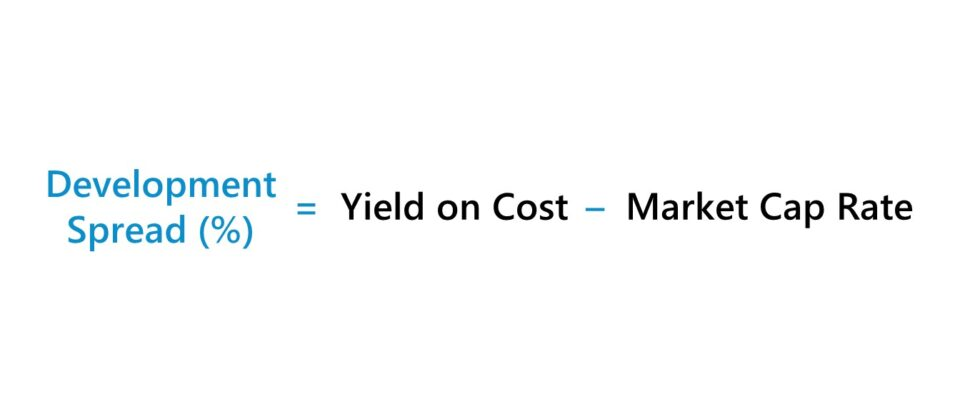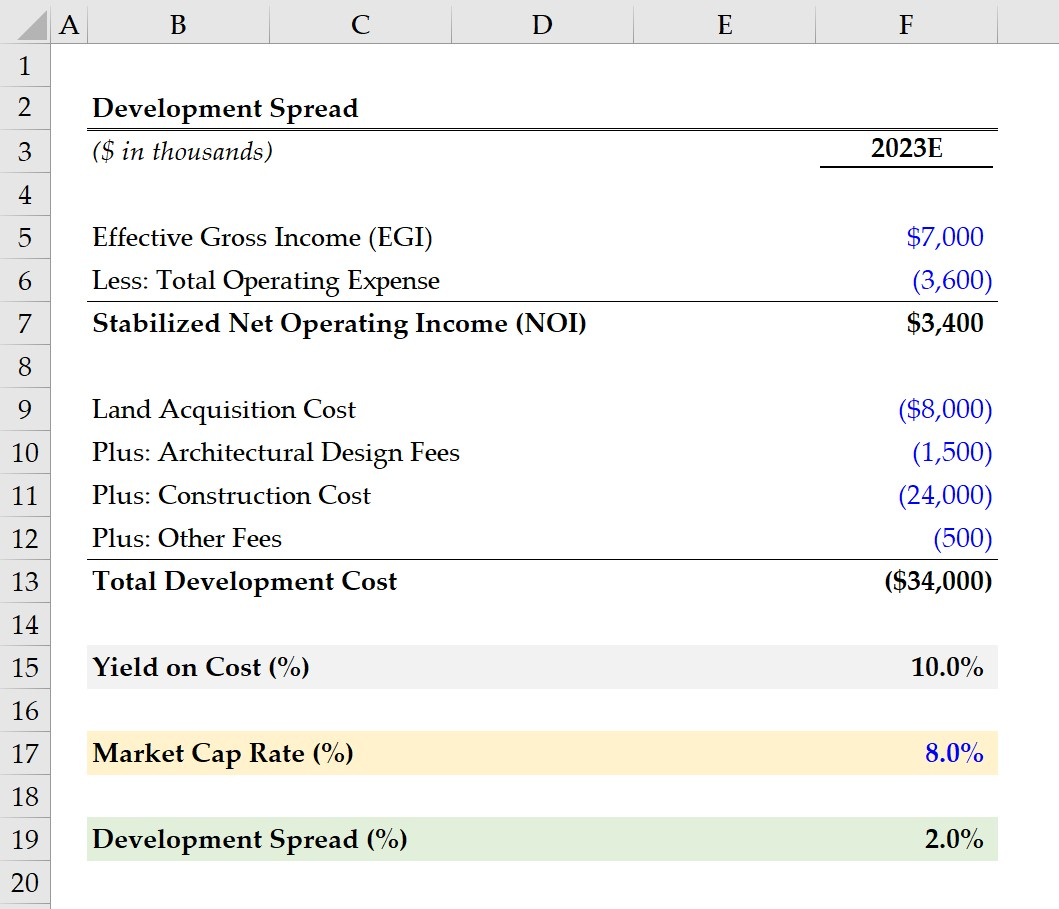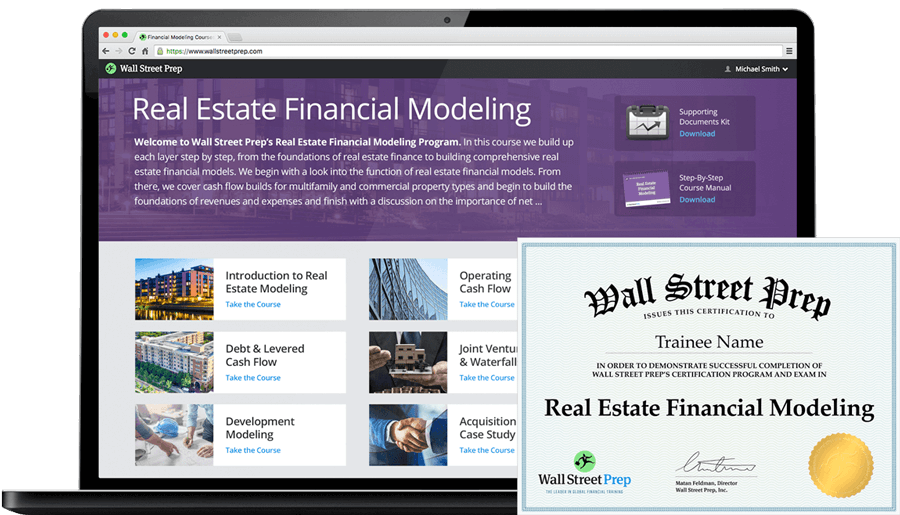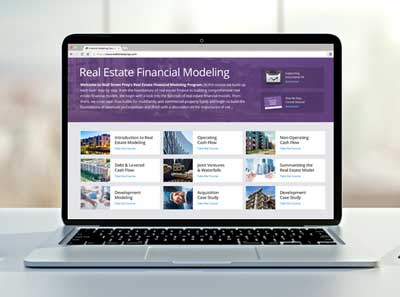Welcome to Wall Street Prep! Use code at checkout for 15% off.Guide to Understanding the Development Spread## How to Calculate Development Spread?

In the real estate sector, the development spread is a “back of the envelope” calculation used to quickly determine the financial viability of a development project.

The development spread is the difference between a property’s yield on cost (YoC) and the market cap rate.

The market cap rate and yield on cost are each pro forma metrics, because the numerator, annual net operating income (NOI), is a forward-looking metric that assumes the property is stabilized, i.e. the property (and its rental units) are functional and operating, and thereby generating income on behalf of the property owner.

Often, the market cap rate is used interchangeably with the term “going-out cap rate”, whereas the yield on cost can be referred to as the “going-in cap rate”.

• Going-Out Cap Rate → The distinction is attributable to the fact that the market cap rate is based on the fair value of the property, i.e. the stage at which construction and property renovations are complete.
• Going-In Cap Rate → On the other hand, the yield on cost (YoC) is based on the total development cost, or initial project cost, which implies the development work has not yet started (or is currently still in the early stages, e.g. architectural design).The formula to calculate the development spread subtracts the market cap rate from the yield on cost (YoC) of a property.

Development Spread (%) = Yield on Cost (YoC) Market Cap Rate

The cap rate and yield on cost are each practical measures of returns in the real estate development market and are frequently relied on to analyze potential property investments.

The formula for the two return metrics is as follows.

Yield on Cost (YoC) = Stabilized Net Operating Income (NOI) ÷ Total Development Cost
Market Cap Rate = Stabilized Net Operating Income (NOI) ÷ Fair Market Value (FMV)

Or, as mentioned earlier, the formula can also be expressed using the going-out cap rate and going-in cap rate.

Development Spread = Going-In Cap Rate Going-Out Cap Rate

## What is a Good Development Spread?

Undertaking a real estate development project is a long-term commitment with numerous moving pieces that can impact the outcome of the project.

Therefore, a real estate developer must ensure the potential return is enough to compensate for the riskiness of the project (and the development spread is one method to confirm that).

Simply put, a development project with a greater development spread is more likely to be perceived as a worthwhile long-term investment, all else being equal.

While there is no set industry benchmark for what constitutes a “good” development spread, per se, most real estate developers target a development spread of around 1.5% to 2.5%.

By measuring the difference in the implied yields on comparable real estate projects, the investor can compare the risk/return profile of each to decide which option seems the most economically viable, i.e. the monetary benefits must outweigh the incurred costs.

For instance, if a development project generates a yield equivalent to that of a value-add acquisition with less risk (i.e. the purchase of an existing property), there is no incentive for a rational investor to proceed with the development project and take on the extra burden of risk.

We’ll now move on to a modeling exercise, which you can access by filling out the form below.Submitting...

## 1. Real Estate Rental Property Assumptions

Suppose a real estate developer is in consideration of an opportunity to purchase land to construct a commercial building and must determine if the implied return is sufficient given the risk profile of the development project.

The estimated development cost of the commercial building is \$34 million, with the following costs.

• Land Acquisition Cost = \$8 million
• Architectural Design Fees = \$1.5 million
• Construction Cost = \$24 million
• Other Fees = \$500k

Based on the real estate firm’s pro forma forecast models – i.e. once the design, planning, and construction are complete and the commercial building’s units are rented out to tenants – the effective gross income (EGI) and total operating expenses are projected to be \$7 million and \$3.6 million, respectively.

• Effective Gross Income (EGI) = \$7 million
• Total Operating Expenses = \$3.6 million

## 2. Yield on Cost Calculation Example (YoC)

Upon subtracting the commercial building’s operating expenses from its effective gross income (EGI), the stabilized net operating income (NOI) comes out to be \$3.4 million.

• Stabilized Net Operating Income (NOI) = \$7 million – \$3.6 million = \$3.4 million

The yield on cost, i.e. the development yield, on the commercial property is 10%, which we determined by dividing the stabilized NOI by the total development cost.

• Yield on Cost (%) = \$3.4 million ÷ \$34 million = 10.0%

## 3. Development Spread Calculation Example

In the final section of our exercise, we must determine the market cap rate (or “going-out cap rate”).

In practice, the market cap rate is approximated by performing in-depth diligence on comparable properties in the same location, including research on the current macroeconomic conditions and local trends.

The real estate developer estimates the market cap rate to be 8.0% based on their internal market data and after completing various methods of research, such as speaking with lenders, brokers, and appraisers.

• Market Cap Rate = 8.0%

In conclusion, we will subtract the yield on cost (YoC) from the market cap rate to arrive at an implied development spread of 2.0%.

• Development Spread (%) = 10.0% – 8.0% = 2.0%20+ Hours of Online Video Training

### Master Real Estate Financial Modeling

This program breaks down everything you need to build and interpret real estate finance models. Used at the world's leading real estate private equity firms and academic institutions.

Inline Feedbacks